# How to☝️ Capitalize First Letters in Google Sheets

To capitalize first letters in Google Sheets, select a blank cell, go to the Formula bar, type the formula =PROPER(A1), and hit Enter

This article will instruct you on how to quickly and easily capitalize letters in various ways in Google Sheets.

Stick around so you don’t miss anything important!

## What Are the Default Capitalization Settings in Google Sheets?

When you create a new spreadsheet in Google Sheets, the default capitalization settings are set to Automatic. This means that all cells will be automatically capitalized according to the following rules:

• Each sentence begins with a capital letter.
• Proper nouns are capitalized (names of people, places, or things).
• All other letters are lowercase unless they appear at the beginning of a sentence.

## How to Capitalize the First Letter of Each Word

Some situations require you to capitalize the first letter of each word in a phrase or sentence. Here’s how you can do that with a simple formula:

Difficulty: Beginner

Time Estimate: 5 seconds

1. Select the cell where you want the result.
2. Navigate to the Formula bar and enter the formula =PROPER(A1), where A1 is the cell with text.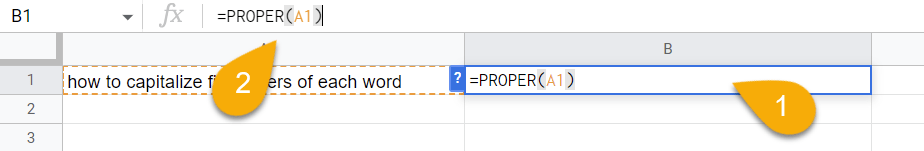1. Press the Enter key on your keyboard.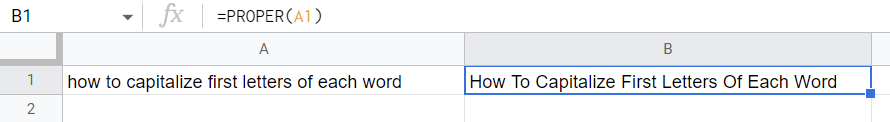There you go! With this formula, each word will be capitalized.

## How to Capitalize Only the First Letter in a Cell

If you just want to capitalize the first letter in a cell, the following steps will show you how:

Difficulty: Beginner

Time Estimate: 7 seconds

1. Click on a blank cell.
2. Go to the Formula bar and enter the formula =REPLACE(A2,1,1,UPPER(LEFT(A2,1))). Here A1 is the cell with text.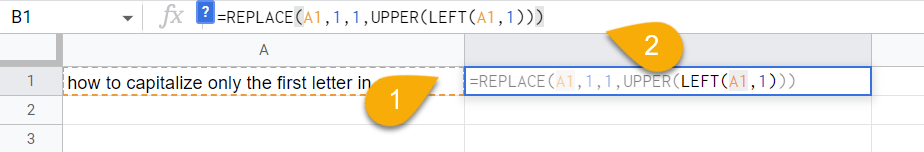1. Hit the Enter key.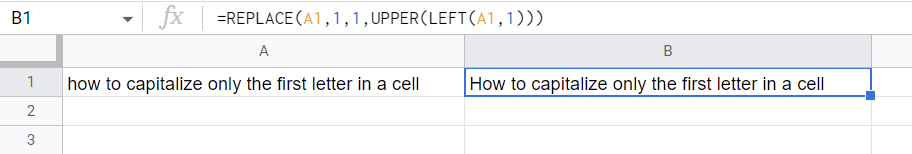Voila! Here is the result!

## How to Capitalize Every Word in a Column

If you want to expand the range of your formula to capitalize all the words in an entire column, check out the description below:

Difficulty: Beginner

Time Estimate: 7 seconds

1. Select a blank cell.
2. In the Formula bar, enter the formula =ARRAYFORMULA(PROPER(A1:A)) where A1:A is the cell range.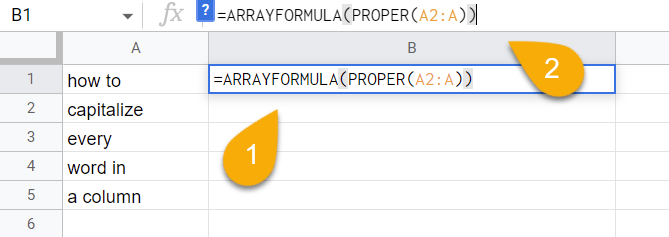1. Press the Enter key on your keyboard.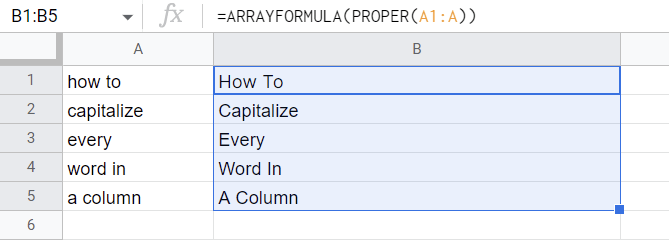It’s as simple as ABC!

## How to Capitalize the First Letter of a Substring

To capitalize the first letter of a string, use the steps illustrated below:

Difficulty: Beginner

Time Estimate: 7 seconds

1. Select the blank cell where you need the result.
2. Navigate to the Formula bar and enter the formula =UPPER(LEFT(A1,1))&RIGHT(A1,LEN(A1)–1). Here A1 contains the text.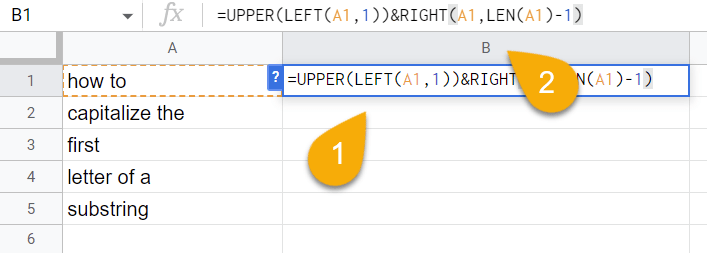1. Hit the Enter key.
2. Drag the icon in the lower right corner of the cell downward to copy the formula for the rest of your list if needed.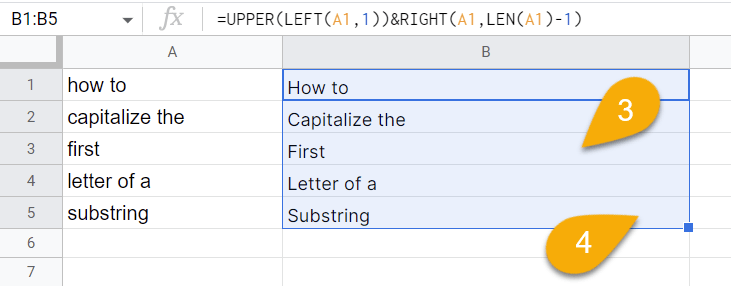That’s all it takes!

## How to Automatically Capitalize First Letters in Google Sheets

If you want to automatically capitalize first letters, use the following solution to do so:

Difficulty: Beginner

Time Estimate: 3 seconds

1. Choose the cell that already contains the result along with the blank cells below it where you also want to apply the formula.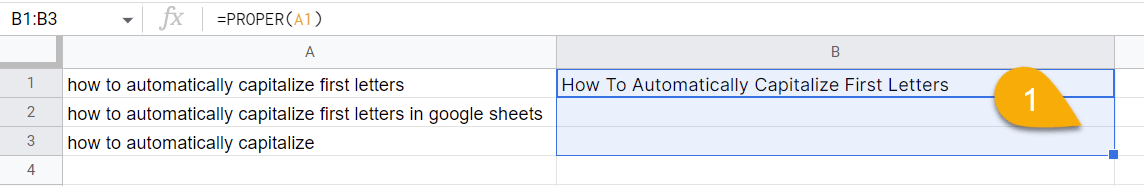1. Press the Ctrl + Enter key combination.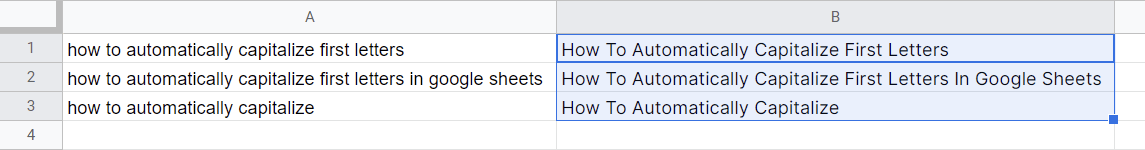Just like that, the formula has been copied for the other cells.

As an alternative, you could select the cell containing the result you have and drag it down to copy the formula to the other cells below it.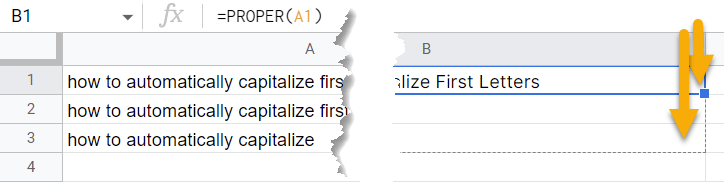Take a look at the result below!